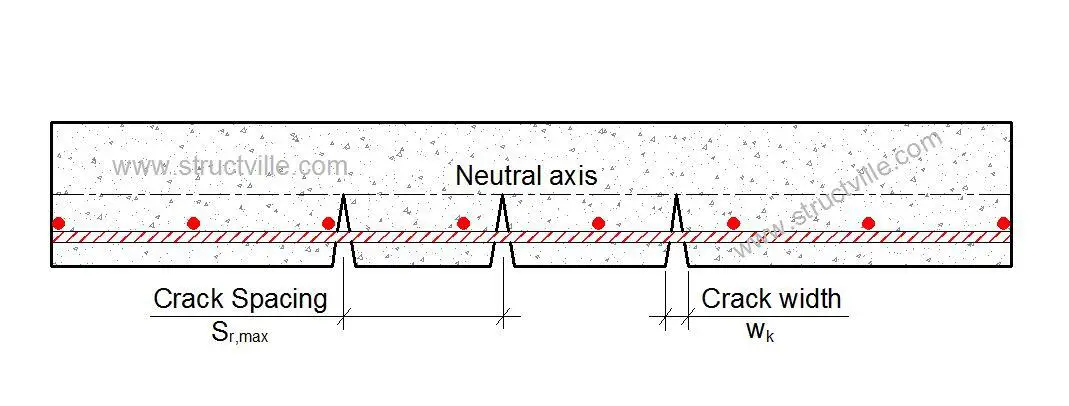# Crack Width and Crack Spacing Calculation in ConcreteCracking occurs in concrete when the restrained strain exceeds the tensile strain capacity of the concrete. In critical elements like water retaining structures, crack control is verified by carrying out direct calculation of the crack width. Crack width is calculated by multiplying the crack inducing strain and the crack spacing (i.e. the movement over a length equal to the crack spacing). This also involves limiting the bar size and/or spacing to recommended limits.

According to expression 7.8 of EN 1992-1-1, crack width wk in a concrete element is given by;

wk = sr,max εcr

where;
sr,max = Maximum crack spacing

sr,max= 3.4c + 0.425 (k1k2ϕ /ρp,eff)

Where;
c = nominal cover, cnom in mm in accordance with BS EN 1992-1

k1 = 0.8 for high-bond bars
(Note that for early age cracking calculations CIRIA C660 suggests a value of 1.14 to account for poor bond conditions, see EN 1992-1-1 for poor bond conditions)

k2 = 1.0 for tension (e.g. from restraint)
= 0.5 for bending
= (ε1 + ε2)/2ε1 for combinations of bending and tension where ε1 is the greater tensile strain at one surface of the section under consideration and ε2 is the lesser tensile strain (i.e. = 0 if strain at second surface is compressive).

ϕ = diameter of the bar in mm.

ρp,eff = As/Ac,eff

This is calculated for each face.

Ac,eff  = min[0.5h; 2.5(c + 0.5ϕ); (h x)/3]

Where;
h = thickness of section
x = depth to neutral axis.

εcr = Crack-inducing strain in concrete

εcr = (εsmεcm)

Crack-inducing strain is derived according to whether the element is subject to:

(1) edge restraint with

• (a) early thermal effects or
• (b) long term effects

(2) end restraint
(3) flexure and/or combinations of flexure and tension from load

Note: It is assumed that the reinforcement will be spaced at reasonably close centres. Where spacing exceeds 5(cnom + ϕ/2), BS EN 1992-1-1 Exp. (7.14) dictates that;

sr,max = 1.3 (h x)

Thank you very much for reading.

Get this publication below for a cheap price by clicking on the image. It goes a long way in supporting what we do here at Structville. To read about the publication, click HERE. Thank you for your kind consideration.